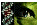Typographical Corrections for Hamer, Mol Vis 2000; 6:265-286.

The typographical corrections below were made to the article on the date noted. These changes have been incorporated in the article and the details are documented here.

28 February 2001:

In the paragraph immediately preceeding Equation 3:

In Eq. 3, nrp is the rate of activation of...

"n" was changed to "ν":

In Eq. 3, νrp is the rate of activation of...

In the paragraph immediately preceeding Equation A3 of Appendix 1:

An ordinary differential equation for PDE; activation may be derived from a simplified enzymatic [19,20]...

the word "reaction" was added:

An ordinary differential equation for PDE; activation may be derived from a simplified enzymatic reaction [19,20]...

In the paragraph immediately preceeding Equation A5 of Appendix 1:

...may be approximated as a pseudo-first-order rate constant, nrp...

"n" was changed to "ν":

...may be approximated as a pseudo-first-order rate constant, νrp...

In the paragraph immediately following Equation A11 of Appendix 1:

where Rectot = Rec* + Rec...

"tot" was made a subscript:

where Rectot = Rec* + Rec...

In the last paragraph of Appendix 1:

The quantity (q5RK*) in Eq. A23 corresponds to the R* decay time constant, (1/tR) in Eqs. A2, A7-8.

"5" was made a subscript and "1/tR" was corrected to "1/τR":

The quantity (q5RK*) in Eq. A23 corresponds to the R* decay time constant, (1/τR) in Eqs. A2, A7-8.

In the third paragraph of the caption for Figure 2:

The signature qualitative feature to note is that in the "Pre & Post" and "Post" conditions in (but not in the "Pre" and "Late" conditions)...

the word "in" was removed:

The signature qualitative feature to note is that in the "Pre & Post" and "Post" conditions (but not in the "Pre" and "Late" conditions)...

In the third paragraph of the caption for Figure 4:

including the highest Ib where the model slope still exceeded -1.

the equality was added to the condition:

including the highest Ib where the model slope was still >=-1.

In Figure 4D, the solid blue curve largely obscured the solid red curve. Also, the curve for the Cass++ clamp is identified in the caption as a "blue dashed curve," but appeared as a solid blue curve. The image: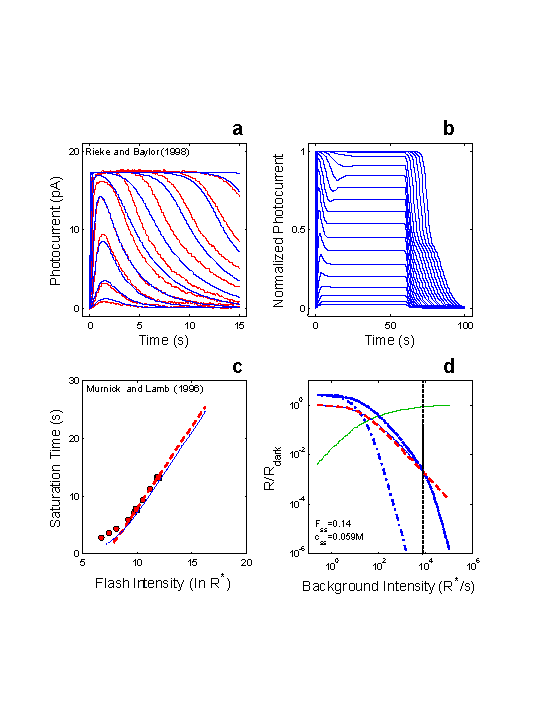was changed to: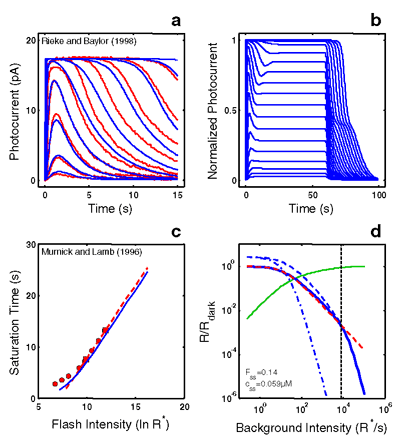In Figure 10D, the solid blue curve largely obscured the solid red curve. The image:was changed to: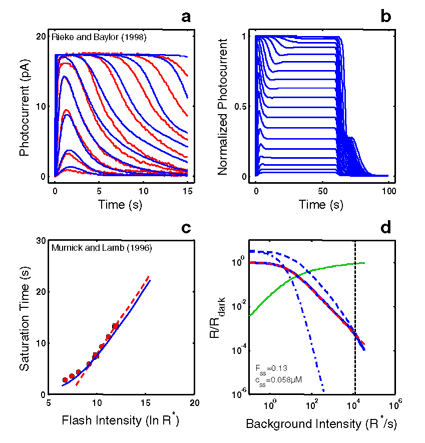In Figure 12D, the solid blue curve largely obscured the solid red curve. The image: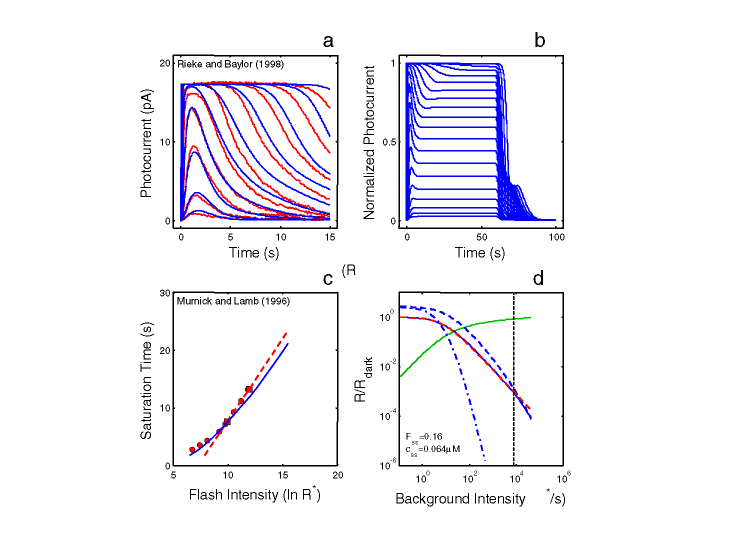was changed to: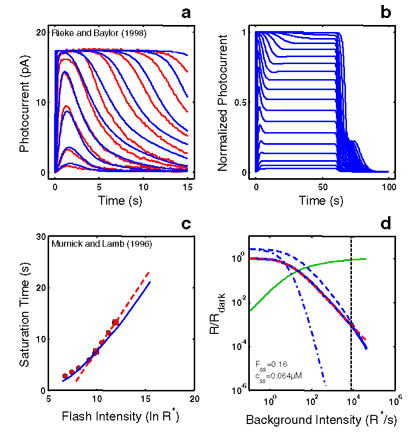In Figure 15D, the solid blue curve largely obscured the solid red curve. The image: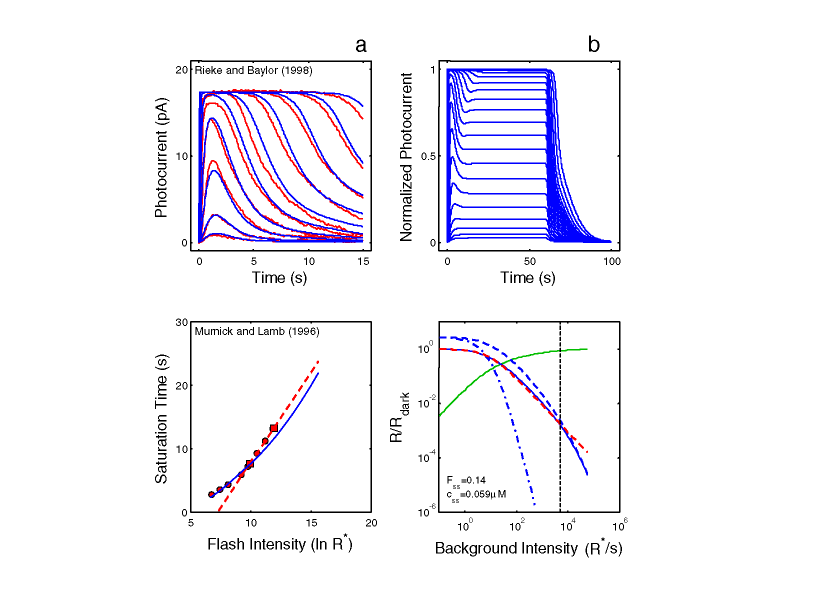was changed to: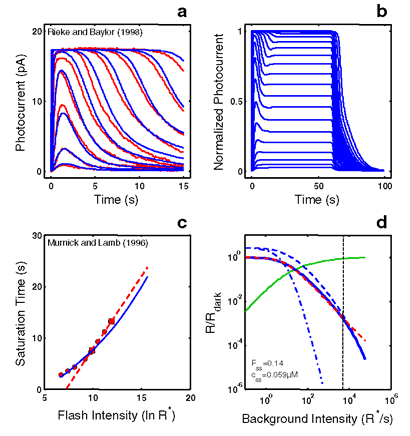The time dependence of F, Rec*, and RK* should not have been shown in the definition of the variables in Table 1. The table: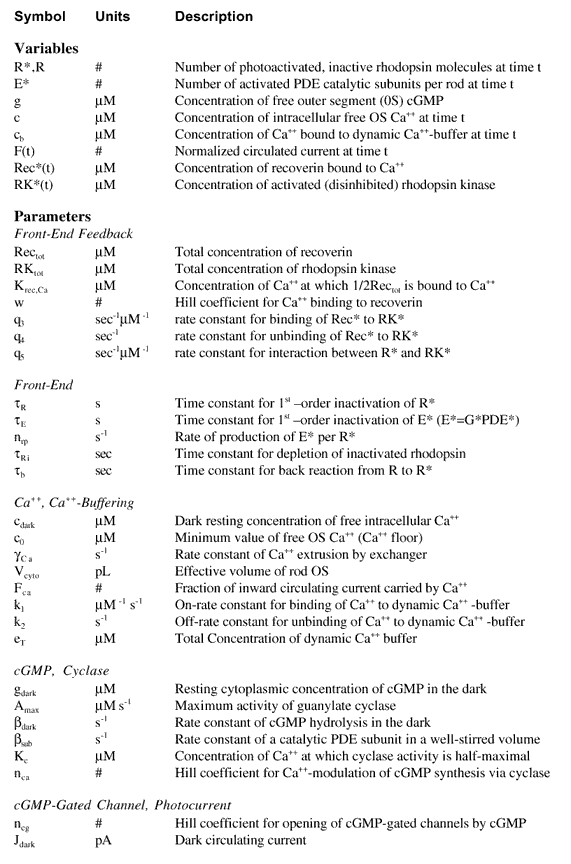was changed to: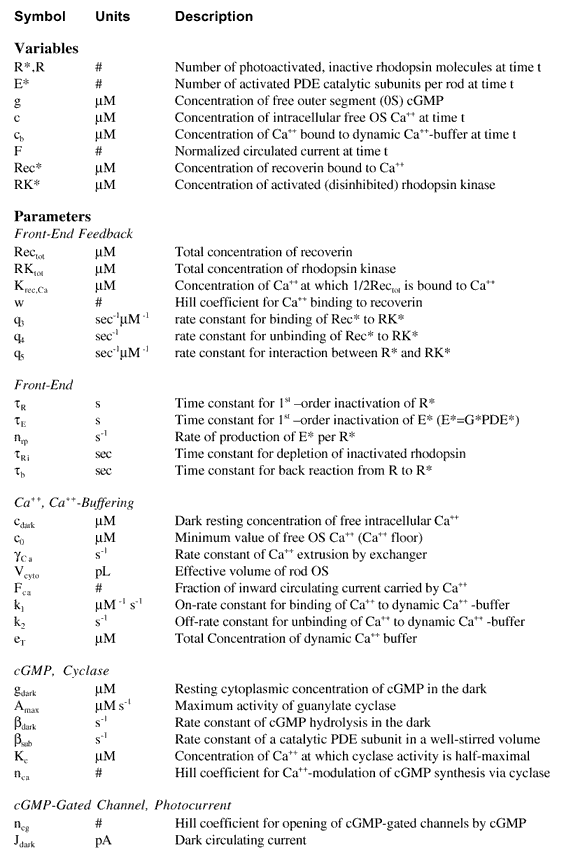In the title of Table 4:

Parameters for Analyses of Combined RecRK-R* activation Model τR Rate Limiting

a colon was added:

Parameters for Analyses of Combined RecRK-R* activation Model: τR Rate Limiting

Hamer, Mol Vis 2000; 6:265-286 <http://www.molvis.org/molvis/v6/a36/>
©2000 Molecular Vision <http://www.molvis.org/molvis/>
ISSN 1090-0535